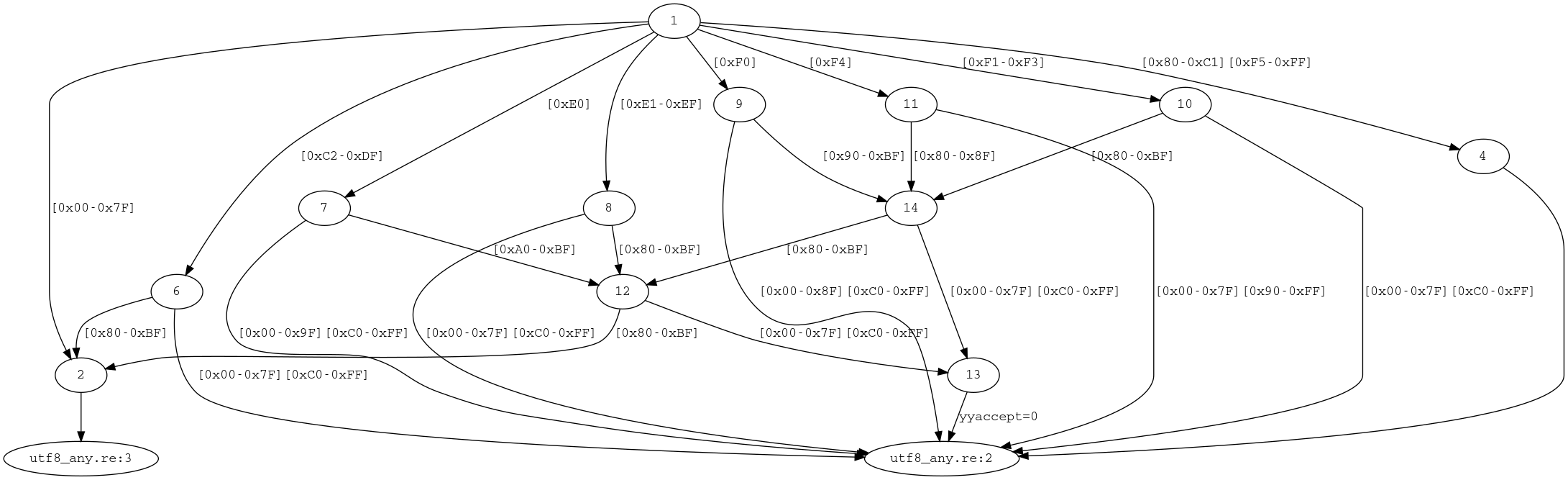# .dot¶

With the `-D, --emit-dot` option, re2c does not generate C/C++ code. Instead, it dumps the generated DFA in the DOT format. One can convert this dump to an image of the DFA using graphviz or another library.

Say we want a picture of the DFA that accepts any UTF-8 code point, `utf8_any.re`:

```/*!re2c
*   {}
[^] {}
*/
```

Generate and render:

```\$ re2c -D -8 -o utf8_any.dot utf8_any.re
\$ dot -Tpng -o utf8_any.png utf8_any.dot
```

Here is the picture:Note that re2c performs additional transformations on the DFA: inserts `YYFILL` checkpoints, binds actions, and applies basic code deduplication. During the transformations, it splits certain states and adds lambda transitions. Lambda transitions correspond to the unlabeled edges on the picture.

A real-world example (JSON lexer, all non-re2c code stripped out), `php_json.re`:

```/*!re2c
re2c:indent:top = 1;
re2c:yyfill:enable = 0;

DIGIT   = [0-9] ;
DIGITNZ = [1-9] ;
UINT    = "0" | ( DIGITNZ DIGIT* ) ;
INT     = "-"? UINT ;
HEX     = DIGIT | [a-fA-F] ;
HEXNZ   = DIGITNZ | [a-fA-F] ;
HEX7    = [0-7] ;
HEXC    = DIGIT | [a-cA-C] ;
FLOAT   = INT "." DIGIT+ ;
EXP     = ( INT | FLOAT ) [eE] [+-]? DIGIT+ ;
NL      = "\r"? "\n" ;
WS      = [ \t\r]+ ;
EOI     = "\000";
CTRL    = [\x00-\x1F] ;
UTF8T   = [\x80-\xBF] ;
UTF8_1  = [\x00-\x7F] ;
UTF8_2  = [\xC2-\xDF] UTF8T ;
UTF8_3A = "\xE0" [\xA0-\xBF] UTF8T ;
UTF8_3B = [\xE1-\xEC] UTF8T{2} ;
UTF8_3C = "\xED" [\x80-\x9F] UTF8T ;
UTF8_3D = [\xEE-\xEF] UTF8T{2} ;
UTF8_3  = UTF8_3A | UTF8_3B | UTF8_3C | UTF8_3D ;
UTF8_4A = "\xF0"[\x90-\xBF] UTF8T{2} ;
UTF8_4B = [\xF1-\xF3] UTF8T{3} ;
UTF8_4C = "\xF4" [\x80-\x8F] UTF8T{2} ;
UTF8_4  = UTF8_4A | UTF8_4B | UTF8_4C ;
UTF8    = UTF8_1 | UTF8_2 | UTF8_3 | UTF8_4 ;
ANY     = [^] ;
ESCPREF = "\\" ;
ESCSYM  = ( "\"" | "\\" | "/" | [bfnrt] ) ;
ESC     = ESCPREF ESCSYM ;
UTFSYM  = "u" ;
UTFPREF = ESCPREF UTFSYM ;
UCS2    = UTFPREF HEX{4} ;
UTF16_1 = UTFPREF "00" HEX7 HEX ;
UTF16_2 = UTFPREF "0" HEX7 HEX{2} ;
UTF16_3 = UTFPREF ( ( ( HEXC | [efEF] ) HEX ) | ( [dD] HEX7 ) ) HEX{2} ;
UTF16_4 = UTFPREF [dD] [89abAB] HEX{2} UTFPREF [dD] [c-fC-F] HEX{2} ;

<JS>"{"                  {}
<JS>"}"                  {}
<JS>"["                  {}
<JS>"]"                  {}
<JS>":"                  {}
<JS>","                  {}
<JS>"null"               {}
<JS>"true"               {}
<JS>"false"              {}
<JS>INT                  {}
<JS>FLOAT|EXP            {}
<JS>NL|WS                {}
<JS>EOI                  {}
<JS>["]                  {}
<STR_P1>CTRL             {}
<STR_P1>UTF16_1          {}
<STR_P1>UTF16_2          {}
<STR_P1>UTF16_4          {}
<STR_P1>UCS2             {}
<STR_P1>ESC              {}
<STR_P1>ESCPREF          {}
<STR_P1>["]              {}
<STR_P1>UTF8             {}
<STR_P1>ANY              {}
<STR_P2>UTF16_1          {}
<STR_P2>UTF16_2          {}
<STR_P2>UTF16_4          {}
<STR_P2>UCS2             {}
<STR_P2>ESCPREF          {}
<STR_P2>["] => JS        {}
<STR_P2>ANY              {}
<*>ANY                   {}
*/
```

Generate .dot file:

```\$ re2c -Dc -o php_json.dot php_json.re
```

Render it with ``dot -Gratio=0.3 -Tpng -o php_json_dot.png php_json.dot``:Render it with ``neato -Elen=4 -Tpng -o php_json_neato.png php_json.dot``:The generated graph is sometimes very large and requires careful tuning of the rendering parameters.